• 2017-06-14 17:11:32

Ax = b 表示一个多项式
A: n * m
x: m * 1
b: n * 1

A的列向量的长度是n，代表一个在n维空间中的向量，m表示有m个这样的向量，
x的各个元素和A的列向量相乘，比如x1 和 A1 相乘表示把向量A1的向量扩展为原来的x1倍，得到一个新的向量，这些向量相加就得到最终的结果b，b也是n维空间的一个向量。

如果希望Ax能表示这个n维空间的所有向量的话，那么m必须大于等于n，就是说，在n维空间中，至少有n个线性无关的向量才能表示。

和多项式的解联系起来，m = n ，而且m个向量线性无关的时候，Ax 正好可以表示一个b，如果 m 小于 n ，那么无解，如果m 大于 n ， 而且A中线性无关的列向量小于n， 那么无解，如果A中线性无关的列向量等于n，那么无穷多解，不存在A中线性无关的列向量大于n的情况。数学
更多相关内容
• 1.多项式的定义： 形如 f（x）= an*x^n+.......ai*x^i+...a0 ，叫做多项式，其中ai 是系数，x 是未知数，i 叫做 指数。 若an 不为0称f（x）位 n 次多项式，记作 deg f（x） 如果 a0 ！=0，且 ai ==0 （i=1.2....n...

1.多项式的定义：

形如 f（x）= an*x^n+.......ai*x^i+...a0 ，叫做多项式，其中ai 是系数，x 是未知数，i 叫做 指数。

若an 不为0称f（x）位 n 次多项式，记作 deg f（x）

如果 a0 ！=0，且 ai ==0 （i=1.2....n）则称f（x）为零次多项式，f（x）=b；等同于K 中非零元

规定 0 多项式 的次数，deg0 = -INF ；

deg(f(x)+g(x))=max(degf(x),deg g(x));

deg(f(x)*g(x)) =degf(x)+deg g(x);

多项式加法满足结合律、交换律，多项式乘法满足交换律与结合律。

2.环的定义。

定义一个非空集合R，如果有两个代数运算，加法与乘法。

那么 a, b 是集合中的两个元。

且 满足加法与乘法，结合律，交换律，分配率，那么称这个集合R是一个环

3.线性空间的定义：

设有一个多项式k[x]可以由

集合 s={1，x, x^2,........x^n}重的有限多个多项式线性表示，任意取s中的一个子集s1={x^i.....x^j};

设 k1x^i+............km*x^j=0;

显然k1...km=0,因此s1是线性无关的，从而 s 线性无关 所以s 是 k【x】的一个基。

从而 k【x】是无限维度的线性空间。

4.一元多项式环

设R是一个环，R对加法封闭，R对乘法封闭。

1>若R 的乘法满足交换律，称 R 是交换环

2>若R中有一个元素e,a是一个元

s.t a*e=e*a =a ，则称 e是 单位元，如果还有单位元，单位元只有一个。

3>如果a 属于 R 如果存在 b 属于R 且 b 不为0 使得 a*b=0；

则称a 是一个左零因子或右乘因子。

特别的 0 成为特别0因子

子环的定义：

如果 对于R的加法和乘法运算也成为一个环，则称环R 的一个非空子集合 R1是R的一个子环，

定义 如果 R1中 任意 元 a 属于 R1，且 -a 属于R1则称R 1是R的一个子环。

同构映射的应用：

这里直接简化来理解：

因为（x+3)^2 =（x^2+6*x+9）

设矩阵A ，单位矩阵 I；

显然 有子环定义 矩阵 矩阵 I是矩阵 A 的一个子环。

那么 （A+3*I）^2= A^2+6*A+9*I(把A 看作x ，I看作常数 1)；

同构映射，一个双映射中元素是一 一对应的，且对加法运算和乘法运算保持封闭

例如：f（x）+g（x）=h（x），f（x）*g（x）=p（x）

定理1

设K是一个 数域，R 是一个由单位环 I 的交换环，且K到R1 有一个同构映射 t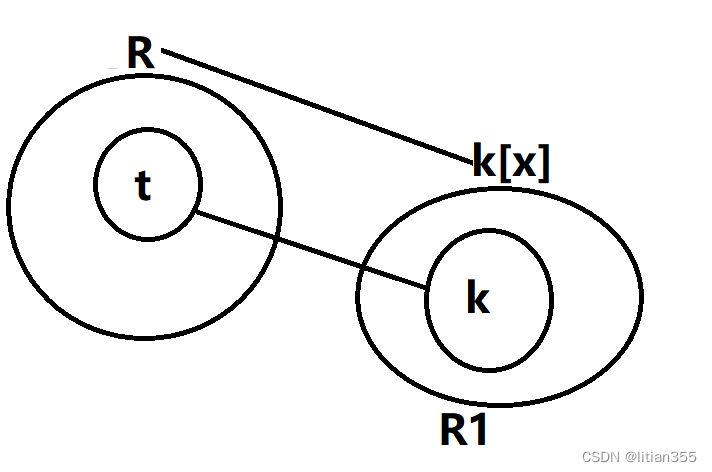上图表示的是任意给 t 属于 R ，令k【x】------>R  的映射

设t 是 f【x】的同构映射，由于 f（x）的表示方法唯一。

通用性质： 设 f（x）+g（x）=h（x），f（x）*g（x）=p（x）；

则   f（t）+g（t）=h（t），f（t）*g（t）=p（t）用t 带入。

展开全文• 在状态空间方程中引入输入和状态的多项式函数, 以此多项式函数表示非线性因素. 为了辨识多项式线性系统中的各系统矩阵, 对于矢量化各系统矩阵组成的未知参数矢量, 分别在无约束和有约束条件下采用两并行分布算法...
• 线性空间是广义的，他的元素可以任何东西，可以是向量，矩阵，多项式，函数……在线性空间选定了基以后就可以表示成向量的形式，这时2者就是同一个意思了。 线性空间的一些直观理解 摘抄于 ...

## 距离

首先我们给出距离的定义。以了解数学上关于距离抽象的定义。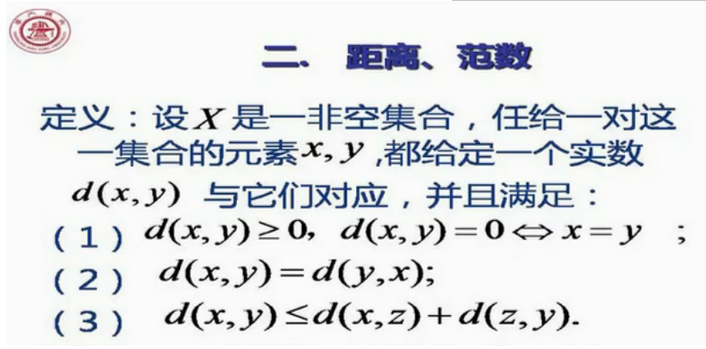如果满足上面的3个条件，我们就称d(x,y)是这两点之间的距离。
另外我们需要理解的是，距离度量的是一种长度，既然距离是长度的量化，那么度量的就有可能是直线也有可能是曲线，就像在地球仪上找2点距离，就需要画一个大圆，然后求弧长。

## 向量间距离

上面讨论的距离，只是一种高度概括抽象的距离的特性。这里给出其中常用的几种具体的向量间距离公式。情形1到情形3，分别是欧氏距离，棋盘距离，曼哈顿距离（也叫城市距离）。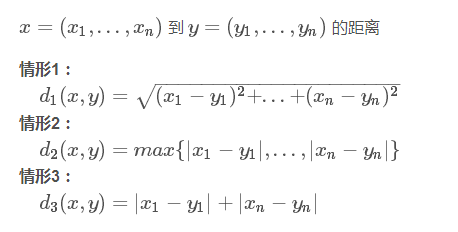## 向量范数

向量范数可以看成向量x=(x1,x2,…,xn)到零点的距离。
当我们这样理解范数的时候，那么对上面3种情形的距离公式，我们得到的对应的向量的范数就为：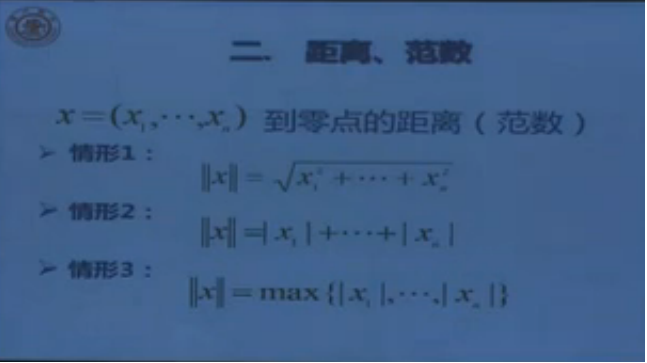我们会发现向量的范数与向量间的距离的公式很相似，即是其中的那个y向量为0时的情况。之所以所有的向量范数都以0向量为标准，是因为在线性空间中0向量是唯一不变的。

# 各种空间的关系

在我们继续展开讨论之前，我希望给出一个总括性的结论，让大家对数学上提出的各种空间的相互关系有一个总的认识。线性空间（向量空间）是一个比较初级的空间，如果在里面定义了范数，就成了赋范线性空间。赋范线性空间满足完备性，就成了巴那赫空间；赋范线性空间中定义了角度，就有了内积空间，内积空间再满足完备性，就得到希尔伯特空间。这些空间都是线性空间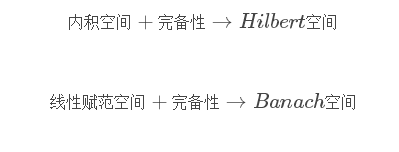那么什么是完备性呢？
如果我们想研究收敛性（极限）怎么办？—定义完备性
简单的说就是空间在极限运算中，取极限不能跑出去。所以，显然有理数集，无理数集不具有完备性。实数集具有完备性。

## 各空间之间差别的简化展示

1.线性空间（向量空间）
线性空间又称作向量空间，关注的是向量的位置，对于一个线性空间，知道基（相当于三维空间中的坐标系）便可确定空间中元素的坐标（即位置）；线性空间只定义了加法和数乘运算。
如果我们想知道向量的长度怎么办？—-定义范数，引入赋范线性空间
2.赋范线性空间定义了范数的线性空间！！
如果我们想知道向量的夹角怎么办？—-定义内积，引入内积空间
3.内积空间定义了内积的线性空间！！
4.欧式空间定义了内积的有限维实线性空间！！
如果我们想研究收敛性（极限）怎么办？—-定义完备
5.Banach空间完备的赋范线性空间！！！
6.Hilbert空间完备的内积空间！！！（极限运算中不能跑出度量的范围）

## 线性空间

线性空间又称作向量空间，关注的是向量的位置，对于一个线性空间，知道基（相当于三维空间中的坐标系）便可确定空间中元素的坐标（即位置）；线性空间只定义了加法和数乘运算。
如果我们想知道向量的长度怎么办？—定义范数，引入赋范线性空间。即在线性空间中，我们是无法得出向量的长度的。

### 向量空间和线性空间的细微区别

线性空间和向量空间基本上是一个东西，但是线性空间中的元素可以是任何东西；在选定基以后可以表示成向量的形式，所以线性空间也叫向量空间。
具体来说，向量空间是狭义的，他的元素只能是向量。线性空间是广义的，他的元素可以任何东西，可以是向量，矩阵，多项式，函数……在线性空间选定了基以后就可以表示成向量的形式，这时2者就是同一个意思了。

### 线性空间的一些直观理解

摘抄于http://blog.csdn.net/soudog/article/details/2050632，感谢作者。
在线性空间中，当你选定一组基之后，不仅可以用一个向量来描述空间中的任何一个对象，而且可以用矩阵来描述该空间中的任何一个运动（变换）。而使某个对象发生对应运动的方法，就是用代表那个运动的矩阵，乘以代表那个对象的向量。
简而言之，在线性空间中选定基之后，向量刻画对象，矩阵刻画对象的运动，用矩阵与向量的乘法施加运动。或者我们也可以把矩阵理解为映射，一种变换函数，通过矩阵的乘法将原始点映射到想要映射的终点。
需要注意的是，上面的一句话中的运动并不是在说真正意义上的运动，即它所造成的结果（形成映射的终点）的过程并不会经过这个线性空间中的任何一个无关点，即不会形成路径到达终点。所以矩阵刻画对象的运动，用矩阵与向量的乘法施加运动改成矩阵是线性空间里跃迁的描述更为准确。

### 基

基是一组线性无关的內积为0的向量的集合。
直观地理解，选基就是找坐标系。

### 相似矩阵

若矩阵A与B是同一个线性变换的两个不同的描述（之所以会不同，是因为选定了不同的基，也就是选定了不同的坐标系），则一定能找到一个非奇异矩阵P，使得A、B之间满足这样的关系：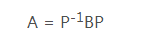上面的公式也就是相似矩阵的定义公式。
从这里看，所谓的相似矩阵，就是同一个线性变换在不同基上的描述矩阵。也就是说一族相似矩阵都是对同一个线性变换在不同基下的描述。

## 赋范空间 （赋范线性空间）

首先给出百度百科上关于赋范空间的定义。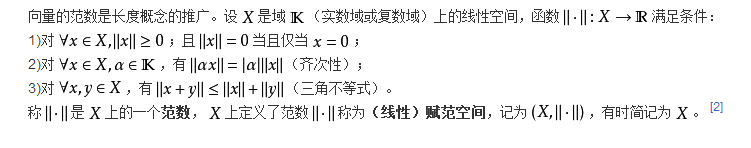从上面的定义可以看出，赋范空间中添加了范数这一概念。所以导致添加了范数的线性空间形成了一个新的空间叫做赋范空间。因此赋范空间有向量的模长，即范数。也就是可以讨论长度了。但是还缺乏一个很重要的概念——两个向量的夹角，为了克服这一缺陷，我们引入了內积的概念。

## 內积空间

内积空间是增添了一个额外的结构的向量空间。这个额外的结构叫做内积，或标量积，或点积。这个增添的结构允许我们严格地讨论矢量的“夹角”和“长度”

展开全文內积空间
• 一.线性变换的不变子空间与Hamilton−CayleyHamilton-CayleyHamilton−Cayley定理 1.不变子空间 (1)概念: (2)性质与判定: 命题1:VVV上线性变换ᎯᎯᎯ的核与象,ᎯᎯᎯ...推论1:设ᎯᎯᎯ是域FFF上线性空间VVV上的线性

一.不变子空间与 H a m i l t o n − C a y l e y Hamilton-Cayley 定理(9.5)1.不变子空间
(1)概念:(2)性质与判定:

命题1: V V 上线性变换 Ꭿ Ꭿ 的核与象, Ꭿ Ꭿ 的特征子空间都是 Ꭿ − Ꭿ- 子空间命题2:设 Ꭿ , B Ꭿ,ℬ 都是 V V 上的线性变换,如果 Ꭿ , B Ꭿ,ℬ 可交换,那么 K e r   B , I m   B , B Ker\,ℬ,Im\,ℬ,ℬ 的特征子空间都是 Ꭿ − Ꭿ- 子空间推论1:设 Ꭿ Ꭿ 是域 F F 上线性空间 V V 上的线性变换, f ( x ) ∈ F [ x ] f(x)∈F[x] ,则 K e r   f ( Ꭿ ) , I m   f ( Ꭿ ) , f ( Ꭿ ) Ker\,f(Ꭿ),Im\,f(Ꭿ),f(Ꭿ) 的特征子空间都是 Ꭿ − Ꭿ- 子空间命题3: V V 上线性变换 Ꭿ Ꭿ 的不变子空间的和与交仍是 Ꭿ Ꭿ 的不变子空间命题4:设 Ꭿ Ꭿ 是域 F F 上线性空间 V V 上的1个线性变换, W = < α 1 , α 2 . . . α s > W=<α_1,α_2...α_s> V V 的1个子空间,则 W W Ꭿ − Ꭿ- 子空间当且仅当 Ꭿ α i ∈ W   ( i = 1 , 2... s ) Ꭿα_i∈W\,(i=1,2...s)命题5:设 Ꭿ Ꭿ 是域 F F 上线性空间 V V 上的1个线性变换, ξ ∈ V \xi∈V ξ ≠ 0 \xi≠0 ,则 < ξ > <\xi> Ꭿ − Ꭿ- 子空间当且仅当 ξ \xi Ꭿ Ꭿ 的1个特征向量(3)将线性变换限制到不变子空间或其商空间上:2.用不变子空间研究线性变换的矩阵表示(1)将线性变换表示成分块上三角矩阵:

定理1:设 A \mathcal{A} 是域 F F n n 维线性空间 V V 上的1个线性变换, W W A \mathcal{A} 的1个非平凡的不变子空间 W W 中取1个基 α 1 . . . α r α_1...α_r ,把它扩充成 V V 的1个基 α 1 . . . α r , α r + 1 . . . α n α_1...α_r,α_{r+1}...α_n ,则 A \mathcal{A} 在此基下的矩阵 A A 为1个分块上三角矩阵 A = [ A 1 A 3 0 A 2 ] A=\left[\begin{matrix}A_1&A_3\\0&A_2\end{matrix}\right] 其中 A 1 A_1 A   ∣   W \mathcal{A}\,|\,W W W 的1个基 α 1 . . . α r α_1...α_r 下的矩阵, A 2 A_2 A \mathcal{A} 诱导的商空间 V / W V/W 上的线性变换 A ~ \tilde{\mathcal{A}} V / W V/W 的1个基 α r + 1 + W . . . α n + W α_{r+1}+W...α_n+W 下的矩阵;设 A , A   ∣   W , A ~ \mathcal{A},\mathcal{A}\,|\,W,\tilde{\mathcal{A}} 的特征多项式分别为 f ( λ ) , f 1 ( λ ) , f 2 ( λ ) f(λ),f_1(λ),f_2(λ) ,则 f ( λ ) = f 1 ( λ ) f 2 ( λ ) f(λ)=f_1(λ)f_2(λ)定理2:设 A \mathcal{A} 是域 F F n n 维线性空间 V V 上的1个线性变换,如果 A \mathcal{A} V V 的1个基 α 1 . . . α r , α r + 1 . . . α n α_1...α_r,α_{r+1}...α_n 下的矩阵 A A 为分块上三角矩阵 A = [ A 1 A 3 0 A 2 ] A=\left[\begin{matrix}A_1&A_3\\0&A_2\end{matrix}\right] W = < α 1 . . . α r > W=<α_1...α_r> ,那么 W W A \mathcal{A} 的1个非平凡不变子空间,且 A   ∣   W \mathcal{A}\,|\,W W W 的1个基 α 1 . . . α r α_1...α_r 下的矩阵是 A 1 A_1(2)将线性变换表示成分块对角矩阵:

定理3:设 A \mathcal{A} 是域 F F n n 维线性空间 V V 上的1个线性变换,则 A \mathcal{A} V V 的1个基下的矩阵为分块对角矩阵(3)当且仅当 V V 能分解成 A \mathcal{A} 的非平凡不变子空间的直和: V = W 1 ⊕ W 2 ⊕ . . . ⊕ W s V=W_1\oplus W_2\oplus...\oplus W_s ,并且 A i A_i A   ∣   W i \mathcal{A}\,|\,W_i W i W_i 的1个基下的矩阵(3)寻找线性变换的非平凡不变子空间:引理:设 V V 是域 F F 上的线性空间(可以是有限维的,也可以是无限维的), A \mathcal{A} V V 上的1个线性变换,在 F [ x ] F[x] 中, f ( x ) = f 1 ( x ) f 2 ( x ) f(x)=f_1(x)f_2(x) ,且 ( f 1 ( x ) , f 2 ( x ) ) = 1 (f_1(x),f_2(x))=1 ,则 K e r   f ( A ) = K e r   f 1 ( A ) ⊕ K e r   f 2 ( A ) ( 5 ) Ker\,f(\mathcal{A})=Ker\,f_1(\mathcal{A})\oplus Ker\,f_2(\mathcal{A})\qquad(5)定理4:设 V V 是域 F F 上的线性空间(可以是有限维的,也可以是无限维的), A \mathcal{A} V V 上的1个线性变换,在 F [ x ] F[x] 中: f ( x ) = f 1 ( x ) f 2 ( x ) . . . f s ( x ) ( 9 ) f(x)=f_1(x)f_2(x)...f_s(x)\qquad(9) 其中 f 1 ( x ) , f 2 ( x ) . . . f s ( x ) f_1(x),f_2(x)...f_s(x) 两两互素,则 K e r   f ( A ) = K e r   f 1 ( A ) ⊕ K e r   f 2 ( A ) ⊕ . . . ⊕ K e r   f s ( A ) ( 10 ) Ker\,f(\mathcal{A})=Ker\,f_1(\mathcal{A})\oplus Ker\,f_2(\mathcal{A})\oplus...\oplus Ker\,f_s(\mathcal{A})\qquad(10)3.零化多项式:4.哈密顿-凯莱定理(Hamilton-Cayley Theorem)(1)整环上的矩阵:(2) H a m i l t o n − C a y l e y Hamilton-Cayley 定理:

定理5:设 A A 是域 F F 上的 n n 级矩阵,则 A A 的特征多项式 f ( λ ) f(λ) A A 的1个零化多项式,从而域 F F n n 维线性空间 V V 上的线性变换 A \mathcal{A} 的特征多项式 f ( λ ) f(λ) A \mathcal{A} 的1个零化多项式(3)利用 H a m i l t o n − C a y l e y Hamilton-Cayley 定理分解线性变换:二.最小多项式(9.6)1.定义与性质
(1)线性变换的最小多项式:F F 上线性空间 V V 上的线性变换 A \mathcal{A} 的最小多项式通常记为 m ( λ ) m(λ)

(2)线性变换的最小多项式的性质:

命题6:线性空间 V V 上的线性变换 A \mathcal{A} 的最小多项式是唯一的命题7:设 A \mathcal{A} 是域 F F 上线性空间 V V 上的线性变换, F [ λ ] F[λ] 中的多项式 g ( λ ) g(λ) A \mathcal{A} 的零化多项式当且仅当 g ( λ ) g(λ) A \mathcal{A} 的最小多项式 m ( λ ) m(λ) 的倍式命题8:设 A \mathcal{A} 是域 F F 上线性空间 V V 上的线性变换,则 A \mathcal{A} 的最小多项式 m ( λ ) m(λ) A \mathcal{A} 的特征多项式 f ( λ ) f(λ) F F 中有相同的根(但重数可以不同)推论1:设 A \mathcal{A} 是域 F F n n 维线性空间 V V 上的线性变换,域 E ⊇ F E\supe F ,则 A \mathcal{A} 的最小多项式 m ( λ ) m(λ) A \mathcal{A} 的特征多项式 f ( λ ) f(λ) E E 中有相同的根(但重数可以不同)(3)矩阵的最小多项式:命题8’:域 F F n n 级矩阵 A A 的最小多项式 m ( λ ) m(λ) A A 的特征多项式 f ( λ ) f(λ) F F 中有相同的根(但重数可以不同)推论1:相似的矩阵有相同的最小多项式推论2:设 A A 是域 F F 上的 n n 级矩阵,域 E ⊇ F E\supe F ,则 A A 的最小多项式 m ( λ ) m(λ) A A 的特征多项式 f ( λ ) f(λ) E E 中有相同的根(但重数可以不同)(4)矩阵的最小多项式不随域的扩大而改变:命题9:设 A A 是域 F F 上的矩阵,域 E ⊇ F E\supe F ,则如果 m ( λ ) m(λ) A A 的最小多项式,那么把 A A 看成域 E E 上的矩阵,其最小多项式仍是 m ( λ ) m(λ)2.几类特殊线性变换或矩阵的最小多项式
(1)几类特殊的最小多项式:(2)约当块(Jordan Block):

命题10:设 A \mathcal{A} 是域 F F l l 维线性空间 W W 上的线性变换,如果 A = k I + B \mathcal{A}=k\mathcal{I}+\mathcal{B} ,其中 B \mathcal{B} 是幂零指数为 l l 的幂零变换,那么 W W 中存在1个基使得 A \mathcal{A} 在此基下的矩阵 A A A = [ k 1 0 . . . 0 0 0 0 k 1 . . . 0 0 0 0 0 k . . . 0 0 0 . . . . . . . . . . . . . . . . . . . . . 0 0 0 . . . k 1 0 0 0 0 . . . 0 k 1 0 0 0 . . . 0 0 k ] ( 5 ) A=\left[\begin{matrix}k&1&0&...&0&0&0\\0&k&1&...&0&0&0\\0&0&k&...&0&0&0\\...&...&...&...&...&...&...\\0&0&0&...&k&1&0\\0&0&0&...&0&k&1\\0&0&0&...&0&0&k\end{matrix}\right]\qquad(5) 把(5)式中的矩阵称为1个 l l 约当块,记作 J l ( k ) J_l(k) ,其中 k k 是主对角线上的元素;于是 J l ( k ) J_l(k) 的最小多项式是 ( λ − k ) l (λ-k)^l推论1:域 F F 上的 l l 级矩阵 A A 相似于 J l ( k ) J_l(k) 当且仅当 A A 的最小多项式为 ( λ − k ) l (λ-k)^l(3)线性变换在线性空间与不变子空间上的最小多项式间的关系:

定理6:设 A \mathcal{A} 是域 F F 上线性空间 V V 上的线性变换,如果 V V 能分解成 A \mathcal{A} 的一些非平凡不变子空间的直和: V = W 1 ⊕ W 2 ⊕ . . . ⊕ W s ( 6 ) V=W_1\oplus W_2\oplus...\oplus W_s\qquad(6) 那么 A \mathcal{A} 的最小多项式 m ( λ ) m(λ) m ( λ ) = [ m 1 ( λ ) , m 2 ( λ ) . . . m s ( λ ) ] ( 7 ) m(λ)=[m_1(λ),m_2(λ)...m_s(λ)]\qquad(7) 其中 m i ( λ )   ( i = 1 , 2... s ) m_i(λ)\,(i=1,2...s) W j   ( j = 1 , 2... s ) W_j\,(j=1,2...s) 上的线性变换 A   ∣   W j \mathcal{A}\,|\,W_j 的最小多项式; [ m 1 ( λ ) , m 2 ( λ ) . . . m s ( λ ) ] [m_1(λ),m_2(λ)...m_s(λ)] m 1 ( λ ) , m 2 ( λ ) . . . m s ( λ ) m_1(λ),m_2(λ)...m_s(λ) 的最小公倍式推论1:设 A A 是域 F F 上的1个 n n 级分块对角矩阵,即 A = d i a g { A 1 , A 2 . . . A s } A=diag\{A_1,A_2...A_s\} ,设 A j A_j 的最小多项式是 m j ( λ )   ( j = 1 , 2... s ) m_j(λ)\,(j=1,2...s) ,则 A A 的最小多项式 m ( λ ) m(λ) m ( λ ) = [ m 1 ( λ ) , m 2 ( λ ) . . . m s ( λ ) ] m(λ)=[m_1(λ),m_2(λ)...m_s(λ)](4)约当形矩阵(Jordan Matrix):3.用最小多项式研究线性变换的矩阵表示
(1)线性变换可对角化的条件:

定理7:设 A \mathcal{A} 是域 F F n n 维线性空间 V V 上的线性变换,则 A \mathcal{A} 可对角化当且仅当 A \mathcal{A} 的最小多项式 m ( λ ) m(λ) F [ λ ] F[λ] 中能分解成不同的1次因式的乘积推论1:域 F F 上的 n n 级矩阵 A A 可对角化当且仅当 A A 的最小多项式 m ( λ ) m(λ) F [ λ ] F[λ] 中能分解成不同的1次因式的乘积定理7及其推论使许多特殊类型的线性变换和矩阵是否可对角化的判定变得非常简洁

(2)最小多项式与可对角化的线性变换:

命题11:设 V V 是域 F F 上的线性空间,则
V V 上的幂等变换 A \mathcal{A} 一定可对角化
V V 上的幂零指数 l > 1 l>1 的幂零变换 A \mathcal{A} 一定不可对角化
③当域 F F 的特征不等于2时, V V 上的对合变换 A \mathcal{A} 一定可对角化;当域 F F 的特征等于2时,不等于 I \mathcal{I} 的对合变换 A \mathcal{A} 一定不可对角化
④当 F F 是复数域时, V V 上的周期变换一定可对角化命题12:域 F F 是级数 r > 1 r>1 的约当块 J r ( a ) J_r(a) 一定不可对角化;包含级数大于1的约当块的约当形矩阵一定不可对角化推论1:设 A \mathcal{A} 是域 F F n n 维线性空间 V V 上的线性变换,如果 A \mathcal{A} 可对角化,那么对于 A \mathcal{A} 的任意1个非平凡不变子空间 W W 都有 A   ∣   W \mathcal{A}\,|\,W 可对角化(3)最小多项式与不可对角化的线性变换:附录.利用命题12的推论1确定可对角化的线性变换的不变子空间的结构

命题13:设 A \mathcal{A} 是域 F F n n 维线性空间 V V 上的线性变换,如果 A \mathcal{A} 可对角化,且 λ 1 , λ 2 . . . λ s λ_1,λ_2...λ_s A \mathcal{A} 的所有不同的特征值,那么 A \mathcal{A} 的任一非平凡不变子空间 W W W = ( V λ j 1 ∩ W ) ⊕ ( V λ j 2 ∩ W ) ⊕ . . . ⊕ ( V λ j r ∩ W ) W=(V_{λ_{j_1}}∩W)\oplus(V_{λ_{j_2}}∩W)\oplus...\oplus(V_{λ_{j_r}}∩W) 其中 λ j 1 , λ j 2 . . . λ j r λ_{j_1},λ_{j_2}...λ_{j_r} A \mathcal{A} r r 个不同的特征值,并且对于 V λ j i   ( i = 1 , 2... s ) V_{λ_{j_i}}\,(i=1,2...s) ,存在 A \mathcal{A} 的不变子空间 U j r U_{j_r} ,使得 V λ j i = ( V λ j i ∩ W ) ⊕ U λ j i V_{λ_{j_i}}=(V_{λ_{j_i}}∩W)\oplus U_{λ_{j_i}}命题14:设 A \mathcal{A} 是域 F F n n 维线性空间 V V 上的线性变换,则 A \mathcal{A} 可对角化当且仅当 A \mathcal{A} 的特征多项式在包含 F F 的代数封闭域中的全部 n n 个根(重根按重数计算)都在 F F 中,且对于 A \mathcal{A} 的任一不变子空间 W W ,都存在 A \mathcal{A} 的不变子空间作为 W W V V 中的补空间展开全文• 本文主要总结了解决非线性回归问题的机器学习方法，其中包括多项式线性模型、广义线性(GAM)模型、回归树模型、支持向量回归(SVR)模型，每个模型的方法都有其特点。 多项式线性模型和GAM模型侧重于经验风险误差最小...非线性回归问题 GAM模型 回归树模型 SVR模型
• 在有限域上非线性多元多项式的解集可以是任意向量集,在该向量集所属的空间上能够找到最小数量的陪集,并覆盖该向量集,用该最小数量的陪集来线性化原有多项式成为了可能。文章以《有限域上向量子集中的陪集及最小陪集...
•数据挖掘 人工智能
• 针对称重仪表检测电路中输入与输出值之间存在非线性关系的特性，在比较了几种非线性校正方法的基础上，给出了一种易于微处理器实现的正交多项式的非线性校正方法，求取称重仪表检测电路的非线性特征，实现了称重仪表...
• 特征分别与标签之间的关系 分类问题中特征与标签[0,1]或者[-1,1]之间...线性模型与非线性模型处理非线性分布数据 ，线性回归无法拟合出这条带噪音的正弦曲线的真实面貌，只能够模拟出大概的趋势（欠拟合），而决回归 机器学习 算法
• 寻找零化多项式的一种方法 摘自邱维声《高等代数(下)》Chapter 10.1, Page 270 的最小多项式 分块多角化矩阵线
• 多项式回归也称多元非线性回归，是指包含两个以上变量的非线性回归模型。对于多元非线性回归模型求解的传统解决方案，仍然是想办法把它转化成标准的线性形式的多元回归模型来处理。 多元非线性回归分析方程如果自...
• 线性代数核心思想及应用——线性空间篇（知识点总结及例题详解） 本篇主要内容： 1.线性空间及子空间 2.向量的线性关系 3.基、维数、坐标 4.子空间的交与和 5.子空间的直和 6.线性空间的同构矩阵
• 通过三次多项式和五次多项式插值算法分别对关节空间轨迹进行规划,并用MATLAB仿真得到关节运动角度曲线、角速度曲线和角加速度曲线,验证五次多项式较于三次多项式具有角速度、角加速度无突变的特点,为工业机器人在...
• 一，有序齐次单项式空间2阶有序齐次单项式： 由此推广，可以得到d阶的有序齐次单项式： 由上式可知，如果n和d数据过大时，内积的运算量将会非常大。我们观察二阶有序齐次单项式，在H中做内积有： 我们定义函数：...
• 前两篇博客介绍的是线性回归，线性回归的一个问题是有可能出现欠拟合现象，解决欠拟合其中的一个方法是本文的多项式扩展，还有一个是后面的博客会介绍的局部加权线性回归（Locally Weighted Linear Regression，LWLR...
• 讨论了LBMa空间上的单调函数用单调多项式的逼近问题,构造了两个线性且保持单调的算子Sn (f,x )和Ln (f,x ),证明了它们在LBMa空间上有界,并且与f的误差可以用二阶带权连续模控制.
• 本文介绍了线性空间的概念，线性空间又称向量空间，每个线性空间都有对应的基域、零元，支持对应的向量加法和标量乘法。线性空间中的一组向量满足向量加法及标量乘法在组内封闭，且组内包含零向量，则构成线性子空间...人工智能 数学
• 1. 线性空间  1）集合与映射  a）集合：数域  b）映射：定义；变换；运算  2）线性空间及其性质  a）线性空间是某类事物从量的方面的一个抽象  b）线性空间（向量空间）定义：  定义内容；线性...
• 《矩阵论》东南大学公开课随课笔记-线性空间与变换（1）数学 笔记
• 从而得到不同的模型，广义线性模型正式将指数分布族中的所有成员（每个成员正好有一个这样的联系）都作为线性模型的扩展，通过各种非线性的连接函数将线性函数映射到其它空间从而大大扩大了线性模型可解决的问题。...
• 有关线性空间的基、维数、坐标的相关定义，以及引出的一些定理。矩阵 算法 笔记
• 因为核函数的其中一个妙处就在于：我们发现“先求次项到高维再内积”等于“先低维空间内积再求次项”。既然有这么一个现象，肯定是先在低维做容易些。核函数也是这种计算思路的体现，减少了计算量。 高斯核函数 提到...支持向量机 机器学习 人工智能 算法 数学
• 交换定理是线性代数中我最喜欢的定理之一。因为我看了四五遍，才把定理的证明弄明白。...但是，很多国内的线性代数书籍，都只是在实数域和n维实数向量空间上定义线性独立，没有上升到代数学的高度。因此这里还是有...
• 1.在线性空间的理论中，有一个定理起着重要的作用，描述如下,如果向量组 中每一个向量都是向量组 的线性组合，而且m>k,则向量 必定线性相关. 证明过程如下，由题设: 必然存在KxK的满秩矩阵A，使得 ...机器学习 算法
• 文章内容：使用三次多项式对y = sin2πx进行插值。下面只涉及解题思路，不涉及相关概念。 一、解题思路 二、数据处理 三、运行结果 1.三次多项式系数 2.插值结果与y = sin2πx的比较 四、代码实现...机器学习 matlab
• 矩阵分析——线性空间与线性映射（三） 哈工大严老师矩阵分析笔记 命题：（维数的唯一性） 设，……及，……分别是V的两个基则。 定理：基（坐标系）实现了抽象线性空间到标准线性空间之间的一一对应。...机器学习 图像识别 图像处理 深度学习
• 尝试用线性回归模型，来解决欠拟合问题，效果不好 我们可以使用线性回归模型来拟合数据，然而，在现实中，数据未必总是线性（或接近线性）的。当数据并非线性时，直接使用LinearRegression的效果可能会较差，产生欠......